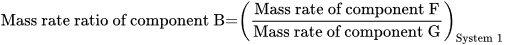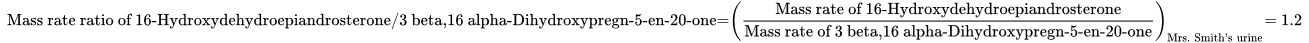Select Page

# Mass rate ratio

### Definition in words

The mass rate of component F divided by mass rate of component G in system 1

Note 1: Component F ≠ Component G (≠ = “not equal to”)

Note 2: Component F ∉ Component G (∉ = “is not an element of”)

Note 3: The quotient of mass rate of component F and mass rate of component G is denoted “mass rate ratio of component B”

### Definition by equation### Systematic terms

Mass rate ratio of component B in system 1

### Result type

Ratio scale
• Value sets are positive numerical values
• Algebraic operations are allowed (a=nb; a≠nb)
Numerical values from 0-∞

### Unit

10-6, 10-3, 1 (one), 103, 106, etc.

Unit, one (1) is omitted in the NPU syntax

### Reference

Ferard G, Dybkaer R, Fuentes-Arderiu X. Compendium of Terminology and Nomenclature of Properties in Clinical Laboratory Sciences : Recommendations 2016. 1 ed: Royal Society of Chemistry; 2016. 182 p.

### Clinical Chemistry

Generic abbreviated form NPU57713 Pt(U)—16-Hydroxydehydroepiandrosterone/3 beta,16 alpha-Dihydroxypregn-5-en-20-one; mass rate ratio = ?
Full form with a result NPU57713 Patient(Urine)—16-Hydroxydehydroepiandrosterone/3 beta,16 alpha-Dihydroxypregn-5-en-20-one; mass rate ratio = 1.2 x one
Abbreviated form with a result NPU57713 Pt(U)—16-Hydroxydehydroepiandrosterone/3 beta,16 alpha-Dihydroxypregn-5-en-20-one; mass rate ratio = 1.2
Written expression The mass rate ratio of 16-Hydroxydehydroepiandrosterone/3 beta,16 alpha-Dihydroxypregn-5-en-20-one in Mr. Smith‘s urine is [1.2].
Equation• # Kind-of-property

• ## Kind-of-quantity

### Reference

• Dybkaer R. Concept system on ‘quantity’: formation and terminology. Accredit Qual Assur. 2013;18(3):253-60.
• Dybkaer R. ISO terminological analysis of the VIM3 concepts ‘quantity’ and ‘kind-of-quantity’. Metrologia. 2010;47(3):127-34.
Date Term Note
2017-06-28 Mass rate ratio Term established

QU104290